• 2017-07-25 17:45:26

# 序列分解

### 1、非季节性时间序列分解

移动平均MA(Moving Average)

①SAM（Simple Moving Average）
简单移动平均，将时间序列上前n个数值做简单的算术平均。
SMAn=(x1+x2+…xn)/n

②WMA(Weighted Moving Average)
加权移动平均。基本思想，提升近期的数据、减弱远期数据对当前预测值的影响，使平滑值更贴近最近的变化趋势。
用Wi来表示每一期的权重，加权移动平均的计算：
WMAn=w1x1+w2x2+…+wnxn

R中用于移动平均的API
install.packages(“TTR”)
SAM(ts,n=10)

• ts 时间序列数据
• n 平移的时间间隔，默认值为10

WMA(ts,n=10,wts=1:n)

• wts 权重的数组，默认为1:n
#install.packages('TTR')
library(TTR)

plot(data$公司A, type='l') data$SMA <- SMA(data$公司A, n=3) lines(data$SMA)

plot(data$公司A, type='l') data$WMA <- WMA(data$公司A, n=3, wts=1:3) lines(data$WMA)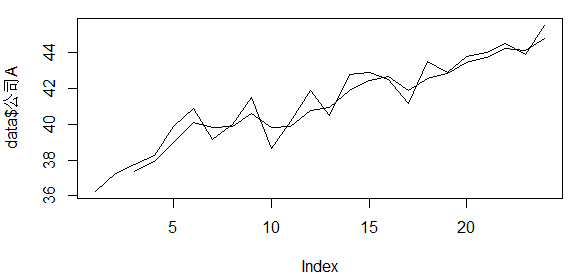### 2、季节性时间序列分解

在一个时间序列中，若经过n个时间间隔后呈现出相似性，就说该序列具有以n为周期的周期性特征。
分解为三个部分：
①趋势部分
②季节性部分
③不规则部分
R中用于季节性时间序列分解的API
序列数据周期确定

• freg<-spec.pgram(ts,taper=0, log=’no’, plot=FALSE)
• start<-which(freq spec==max(freq $spec==max(freq$spec))周期开始位置
• frequency<-1/freq freq[which(freq $freq[which(freq$spec==max(freq$spec))]周期长度 序列数据分解 decompose(ts) data <- read.csv("data2.csv", fileEncoding = "UTF8") freq <- spec.pgram(data$总销量, taper=0, log='no', plot=FALSE);

start <- which(freq$spec==max(freq$spec))
frequency <- 1/freq$freq[which(freq$spec==max(freq$spec))] data$均值 <- data$总销量/data$分店数

freq <- spec.pgram(data$均值, taper=0, log='no', plot=FALSE); start <- which(freq$spec==max(freq$spec)) frequency <- 1/freq$freq[which(freq$spec==max(freq$spec))]

plot(data$均值, type='l') meanTS <- ts( data$均值[start:length(data$均值)], frequency=frequency ) ts.plot(meanTS) meanTSdecompose <- decompose(meanTS) plot(meanTSdecompose) #趋势分解 meanTSdecompose$trend
#季节性分解数据
meanTSdecompose$seasonal #随机部分 meanTSdecompose$random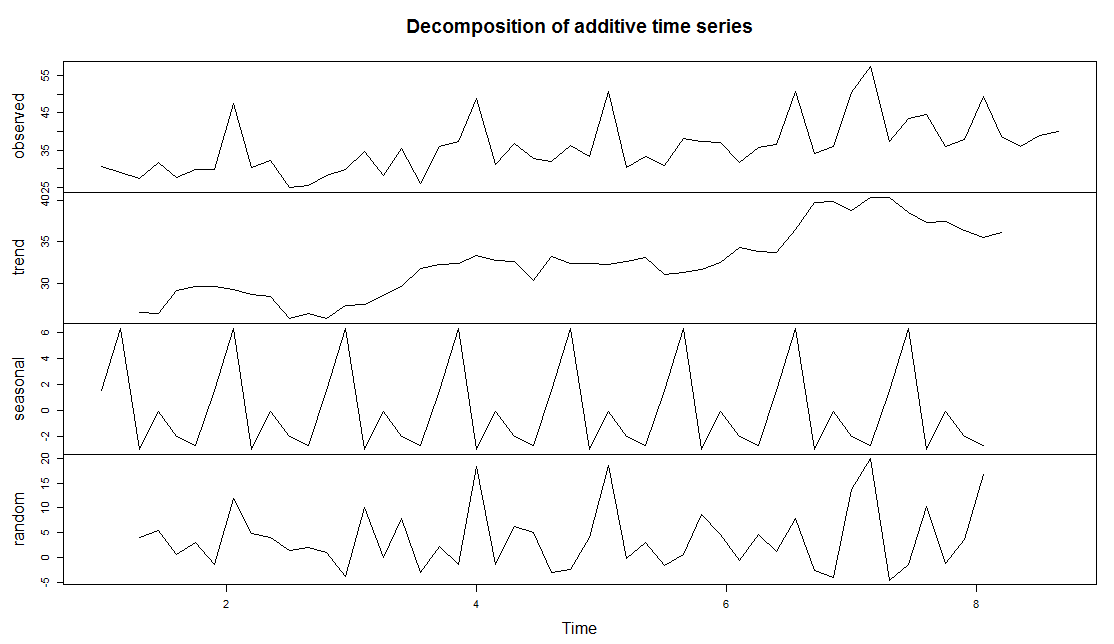R
更多相关内容
•文档
• 0 SARIMAX模型时间序列分析步骤 1.用pandas处理时序数据 2. 检验时序数据的平稳 3. 将时序数据平稳化 4. 确定order 的 p.d.q值 5. 确定season_order的四个值 6.应用SARIMAX模型对时序数据进行预测 其实...

# 0 SARIMAX模型时间序列分析步骤

1. 用pandas处理时序数据

2. 检验时序数据的平稳性

3. 将时序数据平稳化

4. 确定order 的 p.d.q值

5. 确定season_order的四个值

6. 应用SARIMAX模型对时序数据进行预测

其实SARIMAX比ARIMA模型就多了个season_order参数的确定，但也是这里最费时间的一个步骤

# 1 将数据转化成为时序数据

## 先一股脑导入一下工具包

import pandas as pd
import datetime
import matplotlib.pyplot as plt
from pylab import mpl
mpl.rcParams['font.sans-serif']=['SimHei']
import seaborn as sns
import statsmodels.tsa.stattools as ts
import statsmodels.api as sm
from statsmodels.tsa.arima_model import ARIMA
from statsmodels.graphics.tsaplots import plot_acf, plot_pacf
import itertools
import warnings
import numpy as np
from statsmodels.tsa.seasonal import seasonal_decompose
#读取数据
data.index = pd.to_datetime(data['date'])
data.drop(['date'], axis=1, inplace=True)
data = data.result
data.head()
#数据大致情况展示
data.plot(figsize=(12,8))
plt.legend(bbox_to_anchor=(1.25, 0.5))
plt.title('result')
sns.despine()
plt.show()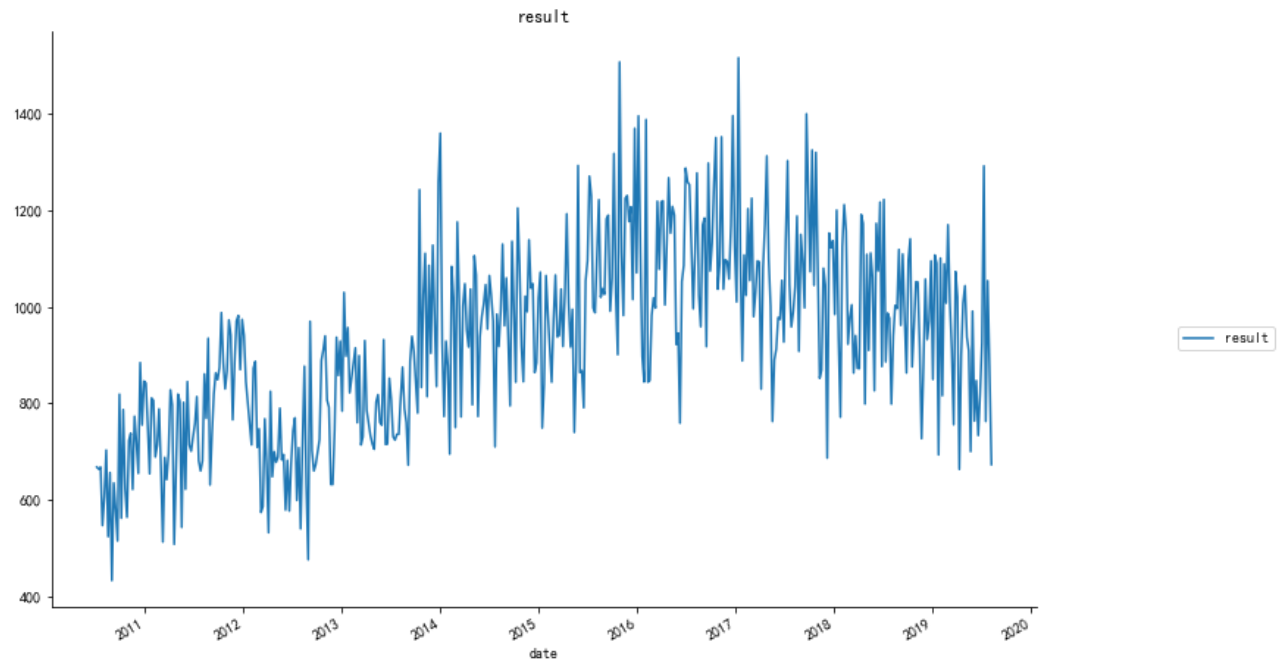# 2 序列平稳性检测

#数据平稳性检测 因为只有平稳数据才能做时间序列分析
def judge_stationarity(data_sanya_one):
print(dftest)
dfoutput = pd.Series(dftest[0:4], index=['Test Statistic','p-value','#Lags Used','Number of Observations Used'])
stationarity = 1
for key, value in dftest.items():
dfoutput['Critical Value (%s)'%key] = value
if dftest > value:
stationarity = 0
print(dfoutput)
print("是否平稳(1/0): %d" %(stationarity))
return stationarity
stationarity = judge_stationarity(data)

# 3 序列平稳化

#若不平稳进行一阶差分
if stationarity == 0:
data_diff = data.diff()
data_diff = data_diff.dropna()
plt.figure()
plt.plot(data_diff)
plt.title('一阶差分')
plt.show()

#再次进行平稳性检测
stationarity = judge_stationarity(data_diff)

# 4 做一下季节性分解看看有没有季节性

#季节性分解
decomposition = seasonal_decompose(data,freq=28)
trend = decomposition.trend
seasonal = decomposition.seasonal
residual = decomposition.resid

plt.figure(figsize=[15, 7])
decomposition.plot()

#季节平稳性检测
stationarity = judge_stationarity(residual)

# 5 对order参数p、q定阶，下面两种都可以，但图我还是看不来，下一种傻瓜式（稍微费时间）

#画ACF图和PACF图来确定p、q值
from statsmodels.graphics.tsaplots import plot_acf, plot_pacf

def draw_acf_pacf(ts,lags):
f = plt.figure(facecolor='white')
plot_acf(ts,ax=ax1,lags=lags)  #lags 表示滞后的阶数，值为30，显示30阶的图像
plot_pacf(ts,ax=ax2,lags=lags)
plt.show()
draw_acf_pacf(myts_diff,30)
#对模型p,q进行定阶
warnings.filterwarnings("ignore") # specify to ignore warning messages
from statsmodels.tsa.arima_model import ARIMA

pmax = int(5)    #一般阶数不超过 length /10
qmax = int(5)
bic_matrix = []
for p in range(pmax +1):
temp= []
for q in range(qmax+1):
try:
temp.append(ARIMA(data, (p, 1, q)).fit().bic)
except:
temp.append(None)
bic_matrix.append(temp)

bic_matrix = pd.DataFrame(bic_matrix)   #将其转换成Dataframe 数据结构
p,q = bic_matrix.stack().idxmin()   #先使用stack 展平， 然后使用 idxmin 找出最小值的位置
print(u'BIC 最小的p值 和 q 值：%s,%s' %(p,q))  #  BIC 最小的p值 和 q 值：0,1

# 6  通过网格搜索对seasonal_order进行定阶

#通过网格搜索对seasonal_order进行定阶,目前就是pdq=011，seasonal_order=2, 2, 1, 52效果比较好，RMSE=202.4582
def get_ARIMA_params(data, pdq, m=12):
p = d = q = range(0, 3)
seasonal_pdq = [(x, x, x, m) for x in list(itertools.product(p, d, q))]
score_aic = 1000000.0
warnings.filterwarnings("ignore") # specify to ignore warning messages
for param_seasonal in seasonal_pdq:
mod = sm.tsa.statespace.SARIMAX(data,
order=pdq,
seasonal_order=param_seasonal,
enforce_stationarity=False,
enforce_invertibility=False)
results = mod.fit()
print('x{}12 - AIC:{}'.format(param_seasonal, results.aic))
if results.aic < score_aic:
score_aic = results.aic
params = param_seasonal, results.aic
param_seasonal, results.aic = params
print('x{}12 - AIC:{}'.format(param_seasonal, results.aic))
pdq = [0, 1, 1]
get_ARIMA_params(data, pdq, m=52)

这上面最关键的是这个m值怎么设定，我设置默认为12。m是季节周期，参考别人代码的时候，月度数据的m为12，那我这里以周为单位，季节周期应该是52吧。一年12个月，52个星期，这个逻辑应该没有问题。

# 7  根据定阶参数进行模型拟合

mod = sm.tsa.statespace.SARIMAX(data,
order=(0, 1, 1),
seasonal_order=(2, 1, 2, 52),
enforce_stationarity=False,
enforce_invertibility=False)
results = mod.fit()
print(results.summary().tables)
results.plot_diagnostics(figsize=(15, 12))
plt.show()

这里模型拟合的时候用的数据的原始数据，而不是差分后的数据，因为order参数中已经设置了d为1，在拟合的时候会自动进行一阶差分，并在预测的时候对预测结果进行差分还原。

# 8  对预测值和真实值作图，并计算RMSE值作为评估参数

predict_ts = results.predict(tpy='levels')  #tpy='levels'直接预测值，没有的话预测的是差值
myts = data[predict_ts.index]  # 过滤没有预测的记录

predict_ts.plot(color='blue', label='Predict',figsize=(12,8))

myts.plot(color='red', label='Original',figsize=(12,8))

plt.legend(loc='best')
plt.title('RMSE: %.4f'% np.sqrt(sum((predict_ts-myts)**2)/myts.size))
plt.show()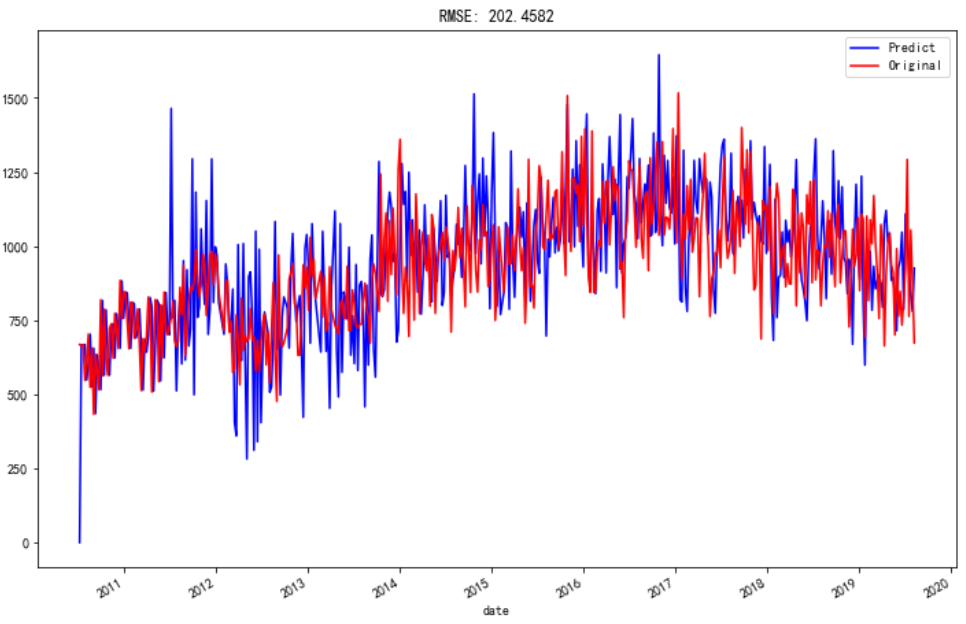# 9 向后对forecast值作图

steps = 20
start_time = myts.index[-1]
forecast_ts = results.forecast(steps)

fore = pd.DataFrame()
fore['date'] = pd.date_range(start=start_time ,periods=steps, freq='7D')
fore['result'] = pd.DataFrame(forecast_ts.values)
fore.index = pd.to_datetime(fore['date'])

predict_ts['2019/1/18':].plot(color='blue', label='Predict',figsize=(12,8))
myts['2019/1/18':].plot(color='red', label='Original',figsize=(12,8))
fore.result.plot(color='black', label='forecast',figsize=(12,8))

plt.legend(loc='best')
plt.show()

这里有个函数pd.date_range是专门用于产生时间序列索引的，start = 开始时间，end = 结束时间，periods=时间索引的个数，freq=‘7D’表示7天为一个时间索引间隔，也可以是'7W'七周，'M'一个月等等。

由于预测的数据没有时间索引，只有序号所以我要在这给他生成时间索引，并合并到dataframe，这样就可以和其他值一起在图像上展示了。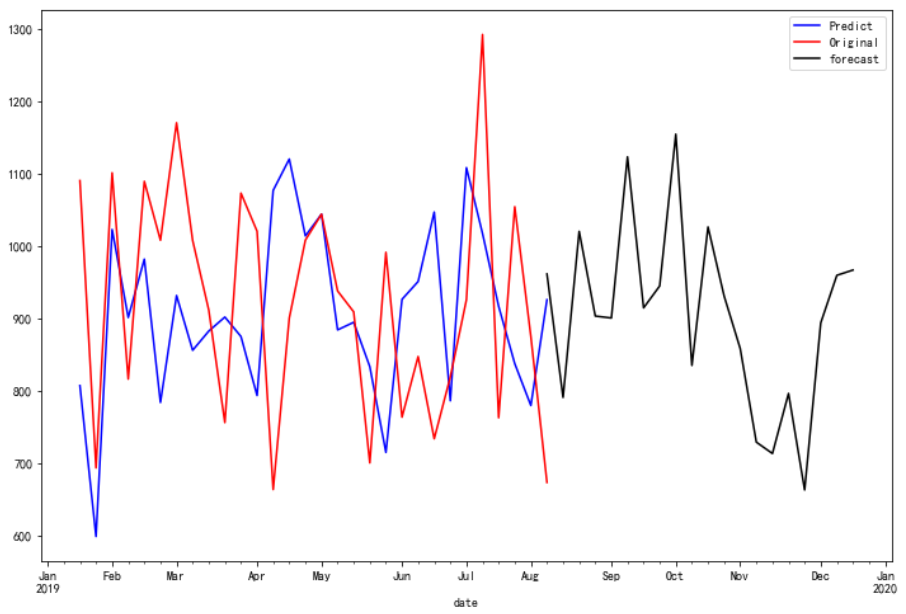最后forecast的效果还是可以的嘛，保存forecast文件

fore.to_csv('forecast_20steps.csv')

展开全文SARIMAX ARIMA
• 本篇以美国1980年-2015年月度失业率为对象，进行一个更为完善的、有季节效应的非平稳时间序列分析流程。 理论支持： 拿到非平稳时间序列，首先进行的就是差分法消除趋势，然后根据情况判断拟合季节加法模型或乘法...

前言：前一篇介绍了对平稳时间序列的分析方法和流程，在没有考虑季节效应的情况下，模型建立的并不成功。本篇以美国1980年-2015年月度失业率为对象，进行一个更为完善的、有季节效应的非平稳时间序列分析流程。
理论支持：
拿到非平稳时间序列，首先进行的就是差分法消除趋势性，然后根据情况判断拟合季节加法模型或乘法模型，最后进行模型检验。常用的模型有两种：ARIMA和因素分解模型。

1. ARIMA(加、乘法)模型，本篇分析采用。
2. 因素分解模型：序列收三个因素影响：长期趋势，季节效应，随机波动。剔除前两者后留下随机波动。使用方法为简单中心移动平均法提取趋势效应，加法乘法提取季节效应。数学运算涉及较多，步骤繁琐，此处不多赘述。

步骤：
一． 数据录入，做出时序图

data unem;
input rate@@;
time=intnx('month','01jan1980'd, _n_ -1);
format time monyy.;
cards;
…………
;
run;
proc gplot data=unem;
plot rate*time/ vaxis=2 to 13 by 0.5;
symbol v=none c=red i=join;
run;初步观察发现，序列有明显的趋势性和周期性。

data unem;
input rate@@;
difrate=dif12(dif(rate));
time=intnx('month','01jan1980'd, _n_ -1);
format time monyy.;
cards;
………
proc gplot;
plot rate*time difrate*time;
symbol v=none c=red i=join;
proc arima data=unem;
run;三． 模型定阶，选择加法还是乘法模型
通常来说，加法模型适用于序列的季节效应，趋势效应和随机波动彼此之间很容易分开。但实践中更常见的情况是，序列的季节效应、长期趋势效应和随机波动之间存在复杂的交互影响关系，简单的加法模型不足以充分提取相关关系，此时应当使用乘法模型。此例中，自相关图显示延迟12阶自相关系数显著大于二倍标准差，说明差分后的序列具有显著的季节效应，此外延迟2、3、5阶也大于二倍标准差，意味着差分后序列还具有短期相关性。在通过加法模型无法充分提取的情况下，我们尝试使用乘法模型，构造原理如下：

1. 用低阶ARMA(p,q)模型提取序列短期相关性。
2. 当序列有季节效应，季节效应本身又具有相关性的时候，用以周期步长为单位的ARMA(P,Q)S 提取季节相关性。
3. 拟合模型实际上为ARMA(p,q)和ARMA(P,Q)S的乘积，记为ARMA(p,d,q) * ARMA(P,D,Q)S

定阶分析过程：
首先观察1阶12步差分后系列12阶以内的自相关系数和偏自相关系数的特征，以确定短期相关模型。自相关图和偏自相关图显示12阶以内均不截尾，考虑使用ARMA(1,1)模型提取短期自相关信息。
再考虑季节相关性，检查延迟12阶、24阶等以周期长度（12）为单位的自相关系数和偏自相关系数的特征。自相关图12阶自相关系数显著，但是24阶系数在二倍标准差范围之内。而偏自相关图显示12阶和24阶都显著，且12阶到24阶之间没有超出二倍标准差范围。因而可以认为季节自相关特征是自相关系数截尾、偏自相关系数拖尾。此时用以12步为周期的ARMA(0,1)12模型提取季节自相关信息。
综上，最终要拟合的乘法模型为ARIMA(1,1,1)*(0,1,1)12。

四． 参数估计
模型定阶之后，参数估计就简单很多了。

/*注释：乘法模型常见格式为ARIMA(p,1,q)*(m,1,n)s, sas拟合命令为：
identify var=x(1,s);
estimate p=(p)(ms)q=(q)(ns)
第一句命令要求系统进行一阶s步差分，这里我们前面差分过了因此不用写。
第二局命令要求系统对差分后序列拟合非季节效应模型ARMA(p,q)与季节效应模型ARMA(m,n)s。合并起来就完成了乘法模型的拟合。*/
estimate p=(1) q=(1)(12) noint;
run;


五． 模型检验

1. 可以发现参数显著性检验通过2. 残差自相关检验中，P值在小于等于延迟24阶时都不大于5%显著性水平，因而不能认为残差序列已经是白噪声序列，即该模型拟合不显著，结合下面残差相关性检验结果进行分析原因。六、问题原因及处理：
传统的纯随机性检验都是借助LB检验统计量进行的，而LB检验统计量是在序列满足方差齐性的假定下构造的。当序列存在异方差属性时，LB统计量不在近似服从卡方分布。所以在条件异方差存在的场合，白噪声检验结果不再准确。通常现象就是残差序列的相关系数很小，近似白噪声序列，但是LB检验结果P值很小。因此，在异方差可能存在的场合，如果自相关系数很小（<0.2），则可以认为残差序列近似为白噪声序列。
残差自回归性检验和异方差性检验：
使用model语句，让系统建立序列关于时间的线性回归模型，检验残差序列5阶延迟的自相关性并输出DW检验的p值，同时对残差序列进行异方差检验:

proc autoreg data=unem;
model difrate=time/nlag=5 dwprob archtest;
run;


DW检验结果，R方很小，模型不显著，证明残差序列不具有显著的自相关性，这与我们之前分析的结果相同（相关系数<0.2，残差序列可以认为是白噪声）。参数估计也表明回归模型不显著，因而总结为残差序列不具有自相关性异方差性(ARCH)检验：图中Q统计量和LM统计量的P值均小于0.05显著性水平，因而可以认定该序列方差非齐。
剩下的步骤为构建GARCH或ARCH模型以及检验，最后预测，内容较多，放在下一篇讨论。

展开全文• 仅需几行代码，您就可以对时间序列进行全面的分析。 该工具箱提供了深入的文档系统和联机帮助，并且其中包含许多演示，这些演示将指导您完成时间序列建模的过程。 Matlab的ECOTOOL工具箱已发布在PLOS ONE中，您可以...
• 时间序列数据的频率高于季度或月度时，许多预测程序在分析季节性影响方面遇到了障碍。 澳大利亚蒙纳士大学的研究人员在美国统计协会杂志（JASA）上发表了一篇有趣的论文，以及一个R程序，以处理这种情况 - 可称为...

# 分析复杂的季节模式

当时间序列数据的频率高于季度或月度时，许多预测程序在分析季节性影响方面遇到了障碍。

澳大利亚蒙纳士大学的研究人员在美国统计协会杂志（JASA）上发表了一篇有趣的论文，来处理这种情况 - 可称为“复杂的季节性”。

我更新并修改了他们的一项计算 - 使用每周而不是每日的汽油价格数据。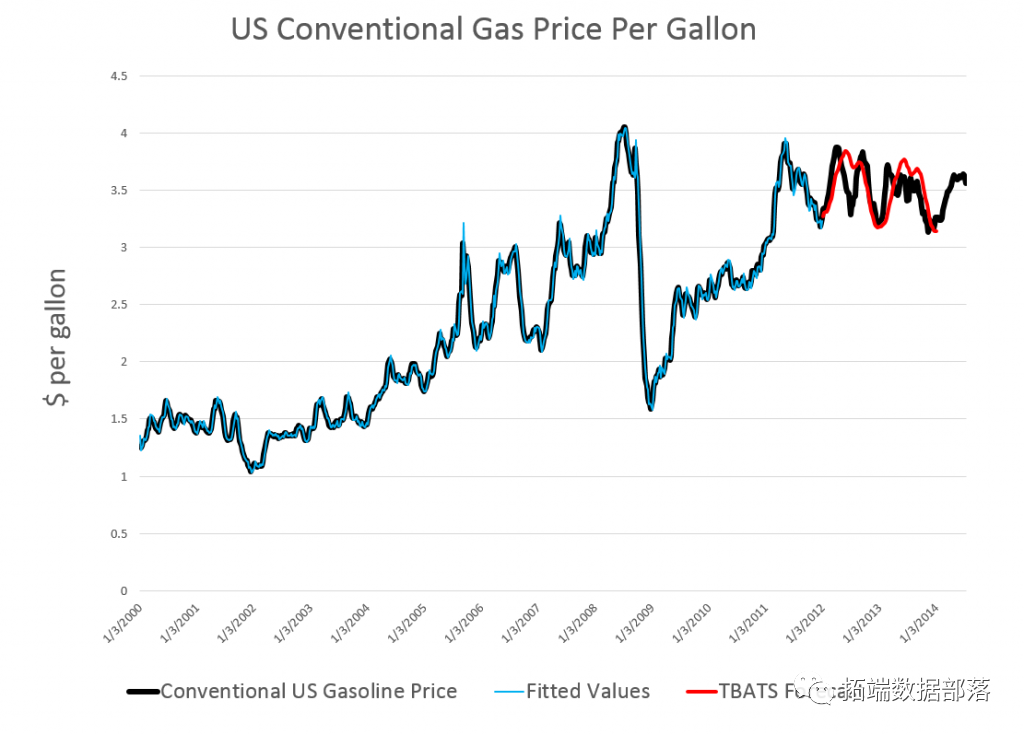我从FRED那里抓取了每周汽油价格。然后，我用2000年到2012年的数据建立了时间序列模型。注意，需要进行一些数据清理工作，将价格数据划分为52周。

104周的预测显示在上图中的红色实线上。

它被称为具有复杂季节性模式的预测时间序列，使用指数平滑以及每日天然气价格，分析日常电力需求。

我只是分析天然气价格数据的一部分，因为我还没有每日数据。但是每周数据中识别的季节性模式很有趣，如下所示。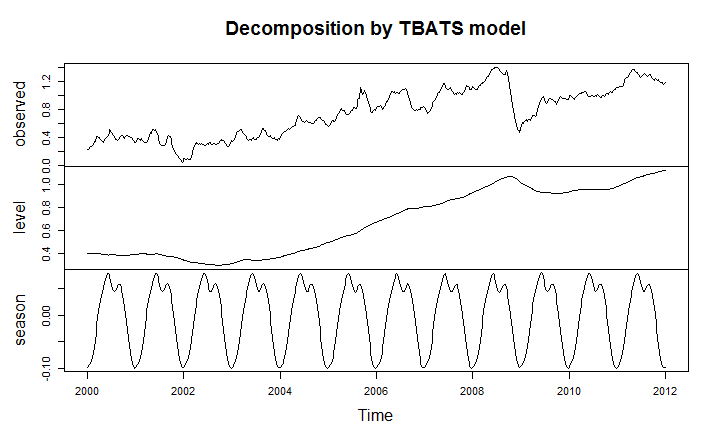从模型的样本外性能来看，这种“季节性波动”在某些情况下可能会更加突出并且非常重要。

适合于较高频率数据的序列提取的季节性模式还具有其他特点，例如估计ARMA（自回归移动平均）模型残差的能力。

当时间序列数据的频率高于季度或月度时，许多预测程序在分析季节性影响方面遇到了障碍。最受欢迎的见解

展开全文R语言
• 在我们的研究中，我们考虑了对印度旁遮普省降雨数据进行统计分析季节性和周期性时间序列模型。 在本研究论文中，我们应用季节性自回归综合移动平均和周期自回归模型来分析旁遮普省的降雨数据。 为了评估模型识别...
• 时间序列分析 我的教授推荐了这本书。 我昨天读和《 。 两者都以自己的方式很好。 前者要先进得多，并提供一些您可能未曾接触过的想法，而后者则是中级的，但是有一些实际的例子，尽管这些例子有些简单化，而且...
• 季节性时间序列数据分析 为什么要进行探索性数据分析？ (Why Exploratory Data Analysis?) You might have heard that before proceeding with a machine learning problem it is good to do en end-to-end analysis...python 大数据 人工智能 机器学习
• 以全国卷烟销量数据为例，用R语言进行时间序列分析，分别建立了ARIMA季节时间序列模型、Holtwinters指数平滑模型，对模型进行了准确评估，附有完整R代码、相关数据集。
• 一.综述 1.概念: "频域分析方法"(Frequency Domain Analysis)又称"(频)谱分析"(Spectral Analysis),是1种非常有用的动态数据...其原理是:假设任何无趋势的时间序列都可以分解为若干不同频率的周期波动.其目标为: ...
•文档
• Java中的时间序列分析。 公告内容 预发行版本0.4现在可用。 项目groupId已更改为com.github.signaflo。 有关更新的坐标，请参见下面的“使用”部分。 特征 季节性ARIMA模型。 ARIMA预测和模拟。 随机游走模拟和...
• SARIMAX (seasonal autoregressive integrated moving average with exogenous regressor)是一种常见的时间序列预测方法，可以分为趋势部分和周期部分；每个部分又可以分为自回归、差分和平滑部分。 趋势稳定...ARIMA
• 运用实际案例讲解如何使用Python编程的方式进行时间序列分析，包括： 自回归移动平均（ARMA）建模方法自回归差分移动平均（ARiMA）建模方法时间序列分解方法（趋势与季节性分析）授课内容简洁明了，突出重点，强调...python 大数据
• matlab拟合差值代码时间序列分析 时间序列是一组有序的点，由一段时间内记录的一些有价值的数据组成。 通常，它以相同长度的连续时间间隔进行计算。 时间序列通常绘制为折线图或散点图，可用于研究记录的数据，预测...
• ## SPSS-季节性分析

千次阅读 2020-10-30 14:31:53
做spss时间序列分析，首先要定义日期变量，只有用spss定义的日期才会被识别为时间序列，其他诸如手动输入日期都是不行的。在spss的data菜单中选择定义日期即可。 来源于此 虚拟机连不上网 打开浏览器-设置-homepage...
• 时间序列季节性分解 可将一个序列分解成一个季节性成分、一个组合趋势和循环成分、一个“误差”成分。 时间序列分两种模型：乘法模型和加法模型，对上面3个分量做乘法或加法。 如果季节性波动的幅度不随着序列水平...矩阵
• 1.季节性时间序列 包含：长期趋势Trend，季节趋势Seasonal，周期循环Circle，随机项Random 这里分解为相加模型X=T+S+C+R   在对时间序列进行分解之前，应该对序列进行检验：（下次写） 2.decompose()函数 将...
• 本篇内容分为两部分：滞后算子和季节性模型，其中介绍前者既回顾了之前的内容，也为介绍后者做了铺垫。1 滞后算子时间序列模型中的自回归项和移动平均项都可以使用滞后算子（Lag Operator...python 人工智能 机器学习 zookeeper
• ARMA是一种平稳时间序列模型，即均值和协方差不随时间的平移而改变。 ARMA有三种类型 AR序列 MA序列 ARMA序列 但是由于ARMA只能处理平稳序列，而现实中的问题往往有趋势或周期等。为了得到平稳序列，我们对...matlab ARIMA
• 之前跟大家一起分享了如何用EXCEL进行回归分析，现在跟大家一起来学习一下如何用EXCEL做时间序列分析。 在百科里，时间序列分析分析的解释是这样的：时间序列（或称动态数列）是指将同一统计指标的数值按其发生的...EXCEL时间序列 时间序列预测 excel预测...